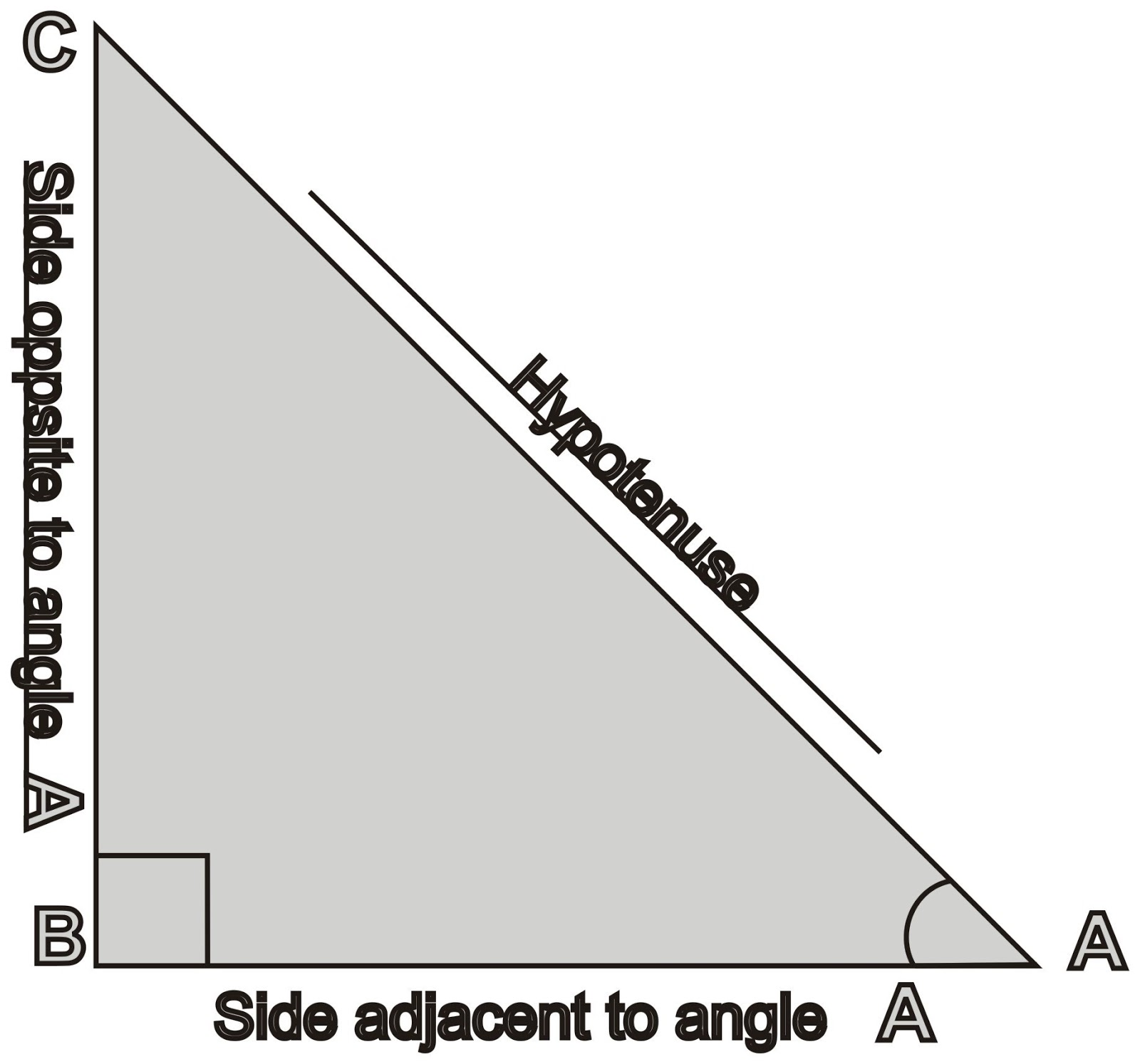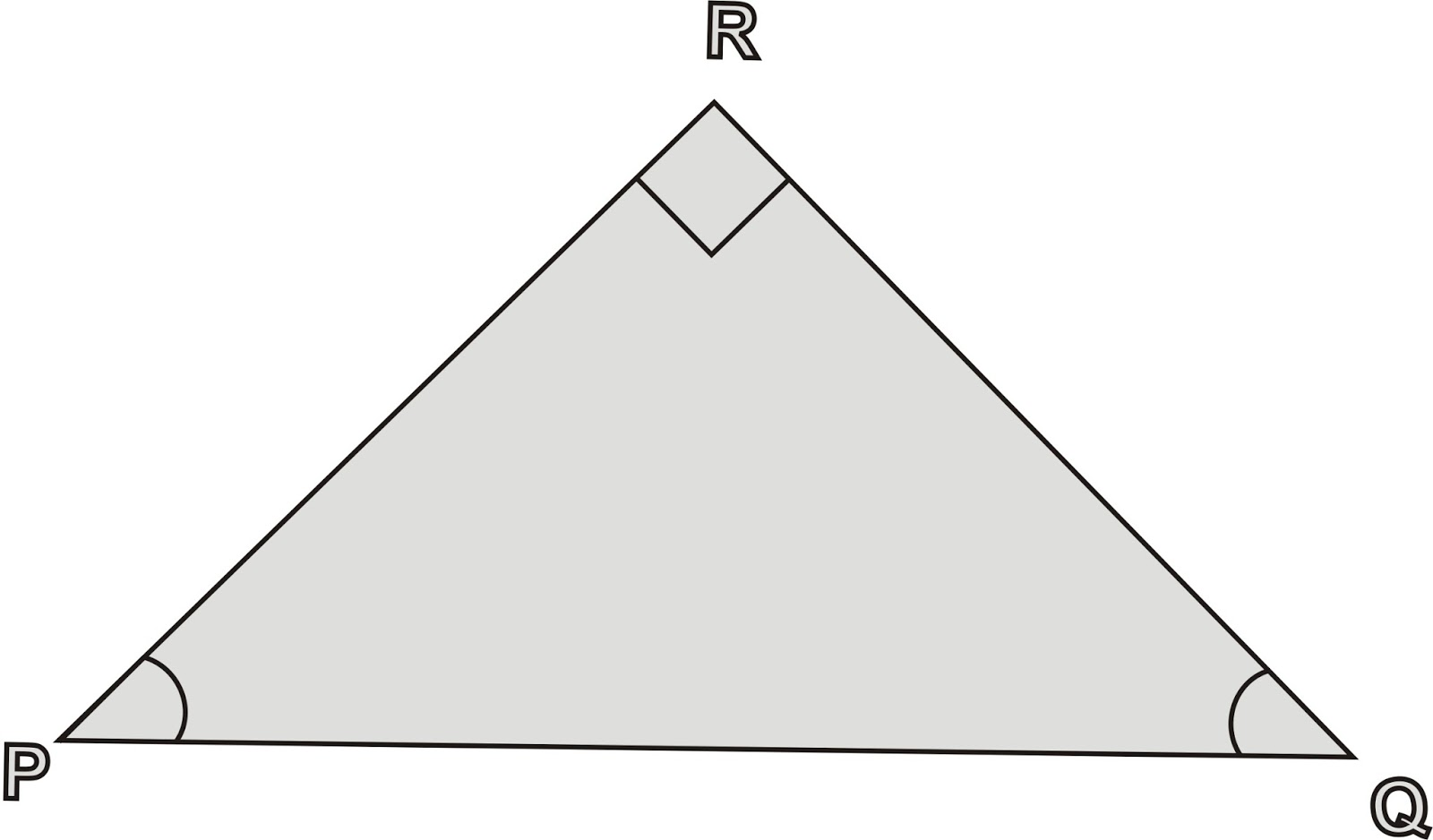## Thursday, September 26, 2013

### 74-Basics of Trigonometry - 02 Important key points

Trigonometry is the important topic for your higher studies. We must study this topic with all basics. Actually, this topic is very simple. If we concentrate to learn this topic from the beginning, we will definitely understand the basics of this topic and it will help us to remember the formulas (formulae) very easily. At the end of all the Blogs related to “Basics of trigonometry”, we will list of all the formulas (formulae) in the separate sheet so that it will be helpful for you to remember all these formulas (formulae).In Blog-82, we asked the following two questions. We are sure that you might get the answers to these questions.  We will see both the answers one by one.

1) Define all trigonometric ratios for an angle C of above triangle ABC.

1) sin C  = AB/AC
2) cos C = BC/AC
3) tan C  = AB/BC
4) csc C = AC/AB
5) sec C  = AC/BC
6) cot C  = BC/AB

## Tuesday, September 17, 2013

### 73-Basics of Trigonometry - 01 Important key points

When an artist wants to draws a picture then he/she always draw the replica of that object. Say, a replica of the great Taj-Mahal of Agra (India) or statue of Liberty and so on. He/She fix the drawing paper on the table. Fixes the distance between paper and his/her eye-site. Then he/she takes all required measurements of the object (Taj-Mahal/Statue of Liberty) on pencil by holding it between his/her eye-site and the object by locating the bottom of an object at the lower side of the pencil and the tip of an object at the tip of the pencil. Using these distances on the pencil he/she fixes the height, width and all other dimensions of an object on the drawing paper. Here, using the properties of similarities in a right-angled triangle, an artist or an architecture

can able to draw the exact replica of an object in the smaller size. He/she holds the tip of the pencil adjusting with the tip of the object and fixes the bottom of the object at the lower part of the pencil to fix the height of an object. In the adjacent figure, an artist/architecture observes an object BC from point A and marking its height as MN on the pencil. Here ABC is the right-angled triangle with AB as the base, BC as the height and AC as hypotenuses. Here the line segments BC and MN are in proportion with AB and AM.
Mathematically, we can say that [BC/AB] = [MN/AM]  .Now let us study something about the right-angled triangle. In the adjacent figure triangle, ABC is the right-angled triangle. < ABC is right-angled, so the side opposite to right angle is known as the hypotenuse. Considering < A is our angle of the right angled triangle, then side AB is known as side adjacent to angle A and the side BC is the side opposite to angle A.

Note: There two types of the concepts of trigonometry. We will consider the first concept as a lower level concept of trigonometry and the other one as the higher level trigonometry. In lower level trigonometry we will study the trigonometric ratios of only the acute angles of the right angled triangle. Where as in higher level trigonometry, we will study the trigonometric ratios for any angle. Now let us start with lower level trigonometry.
Now, let us start our study about trigonometry. For any right angled triangle, there are three sides. Let us write down all possible ratios of the lengths of the triangle.
1) BC/AC
2) AB/AC
3) BC/AB
4) AC/BC
5) AC/AB
6) AB/BC
There are only 6 possible ratios which can be defined from triangle ABC. Considering these 6 ratios, we can develop entire trigonometry. Trigonometric ratios can be defined for certain angle of the right-angled triangle. Let us understand the words "Side opposite to an angle" or "Side adjacent to an angle". See above diagram carefully. In the above diagram, we consider angle A as our angle of right-angled triangle ABC. Side AB is the adjacent to angle A and in the same way side BC is the side opposite to the angle A. We will write above six ratios considering angle A once again using the words "Side opposite to an angle" , "Side adjacent to an angle" and "hypotenuse". We will names these ratios in the trigonometric forms.
1) BC/AC   side opposite to A /  hypotenuse                (sin A)
2) AB/AC   side adjacent to A /  hypotenuse                 (cos A)
3) BC/AB   side opposite to A /  side adjacent to A      (tan A)
4) AC/BC   hypotenuse /  side opposite to A                 (csc A)
5) AC/AB   hypotenuse /  side adjacent to A                 (sec A)
6) AB/BC   side adjacent to A /  side opposite to A      (cot A)

Details of trigonometric ratios:
1) sin A  ------  sine of angle A
2) cos A ------  co-sine of angle A
3) tan A  ------  tangent of angle A
4) csc A ------  co-secant of angle A (generally written as cosec A)
5) sec A ------  secant of angle A
6) cot A ------  co-tangent of angle A

Now we will define all six trigonometric ratios as follows:

1) sin A   =  BC/AC  =  opposite / hypotenuse
2) cos A  =  AB/AC  =  adjacent / hypotenuse
3) tan A   =  BC/AB  =  opposite / adjacent
4) csc A  =  AC/BC  =  hypotenuse / opposite
5) sec A  =  AC/AB  =  hypotenuse / adjacent
6) cot A  =  AB/BC  =  adjacent /  opposite

Now study all basic concepts of trigonometry as discussed above and do at least two experiments as stated bellow.1) Define all trigonometric ratios for an angle C of above triangle ABC .
2) Observe the adjacent figure and write down all the trigonometric ratios.

Anil Satpute

## Monday, September 2, 2013

### 72-Magic Square - Different cell elements and addition

Addition of different cell elements of Magic Squares:

We have seen the addition of the elements in rows columns, and diagonals are the same in all the cases. Each row or column or diagonal contains 4 elements. Now we see the elements in different cases or places will also show the addition as 34.

The following diagram gives us the details of all possible 4 places or cells which we are considering as our elements.(1 + 15 + 12 + 6)      (14 + 4 + 7 + 9)
(R1C1, R1C2, R2C1, R2C2)    (R1C3, R1C4, R2C3, R2C4)